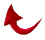IN THE NAME OF ALLAH ALL COMPASIONNET ALL MERCIFUL

Awesome Quranic Mathematical Miracle
"
The Even and the Odd"

May 30, 1991

The Quran’s 19-based mathematical miracle continues to manifest itself in different forms as time goes on. Allah keeps sending reassurance to the believers that the Quran is his unaltered word and no falsehood can enter it.

The miracle that I will be trying to explain to you here is based on a single verse in the Quran which contains only two (Arabic) words! Other translations may show more than two, for example English has 6 words. The verse is Sura 89-Dawn, verse 3. In this verse, Allah swears by "THE EVEN AND THE ODD." At first, this may sound meaningless to the casual reader of the Quran. However, to a sincere student of the Quran it is full of wonders and appreciation for Allah’s power. Let me explain how.

Note that the Sura and verse number is 893 which is a multiple of 19. As a matter of fact, it is 893=47x19. So far in all investigations of the Quran’s mathematical miracle we have not looked at the properties of the integer which is the "quotient", in this case "47". The number 47 happens to also be a "Prime " number (a number which is only divisible by itself and one). The number 47 can be partitioned into 19 + 28 (we subtract the base "19" from 47 leaving 28). 28 is another interesting number mathematically because it is the second "Perfect" number in the number system. The first perfect number is 6. Remember the number of chapters in the Quran is 114 = 6 x 19. What are perfect numbers? A perfect number is a number that is the sum and the multiples of its factors and equals itself. For example, 6 = 1+2+3 and also 6 = 1x2x3. As I mentioned above the second perfect number is 28, and the third 496 and so on. Remember that Allah created the heavens and the earth in "6 Days". He sent the Quran in a PERFECT ARABIC TONGUE. The second perfect number 28 happens to be the number of characters in the Arabic alphabet.

Let me now go back to the EVEN and the ODD. Let us look at the number of verses in each chapter and see if it is odd or even? If we do this we find that there are 60 chapters in the Quran which possess an even number of verses and 54 which possess an odd number of verses (60+54=114). Of course if you add these two numbers you obtain 114 as you should. However, the intricacy of the Quran’s mathematical code becomes more overwhelming if we examine these numbers as the indices of Prime numbers. What do I mean by that? For example the 54th Prime number is 251 and the 60th Prime number is 281. Now let us add them up.

251 + 281 = 532

and

532 = 28 x 19

This is only part of the story! Remember that there are 112 verses in the Quran which are not numbered. These are the Basmalah’s which is in the beginning of every chapter except for chapter 9. What happens if we include these verses in the total number of verses in each chapter? We obtain 52 even numbers and 62 odd numbers. The 52nd Prime number is 239 and the 62nd Prime number is 293. Let’s add them up.

239 + 293 = 532

and

532 = 28 x 19

Glory be to Allah. How is that for a miracle. Do not relax! There is still more! We have two pairs of Prime numbers 251, 281 and 239, 293. Let’s add up all the digits in these two pairs and see what we get!

2 + 5 + 1 + 2 + 8 + 1 = 19

and

2 + 3 + 9 + 2 + 9 + 3 = 28

Again, absolutely awesome! The Almighty has designed the Quran in such a way, which makes it obvious to the sincere that it is His word. Allah willing, by continuous reassurance from Allah, stated in the Quran our faith will increase.

Best regards,

Ali R. FazelyReturn to Home Page